21.1 (EAP/Beta)
20.2
20.1
19.2
19.1
18.2
18.1
17.2

# Diagram Overview

This document details the use of a diagram in the ASP.NET Chart Control: its purpose, implementation and capabilities. Before read this text, it may be useful to review the basic concepts of the chart, which are described in the following document: Charting Basics.

This document consists of the following sections.

## Diagram Overview

The diagram is one of the most basic of a chart’s elements, along the same line as series, chart titles and legend. Architecturally, the diagram is a parent for a chart’s panes and axes, and visually - it encloses a chart’s series as well. Despite the fact that series do not formally belong to the Diagram object (instead, they reside in the WebChartControl’s SeriesCollection), they are stiffly interdependent with the diagram’s type, and are visually displayed within the diagram’s bounds. To view the ASP.NET Chart Control architecture schematically, refer to Chart Elements.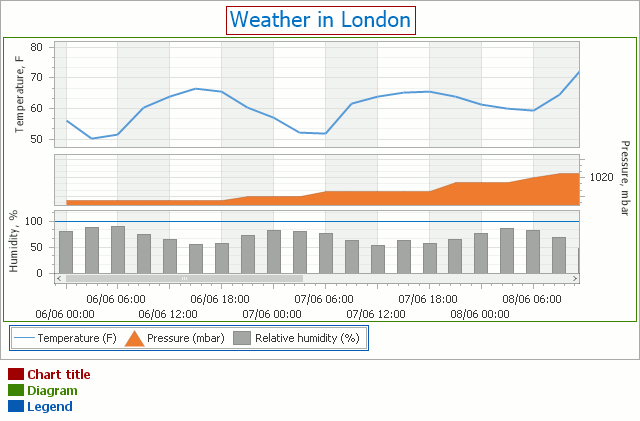Note that it is only possible to have a single diagram object for each chart. However, within the same diagram (of the XY-Diagram type), you can plot multiple panes, as shown in the image above. For details, refer to Panes Overview.

The diagram’s most significant characteristic is its type. The type of the diagram depends on the view type of the chart’s series. By default, it is automatically determined by the view type of the first visible series in the chart’s collection. Note that the Diagram object is equal to null (Nothing in Visual Basic) until a chart has at least one series in its collection.

So, a diagram of each type can only display series of the appropriate view types. And, it is impossible to plot multiple series of incompatible view types within the same Diagram object (and therefore, within the same chart). To learn more on this, refer to Combining Different Series Views.

In addition, for each diagram type there is a unique set of available options and elements.

In the following list, the available diagram types are broken into several basic categories. For specific details on each diagram type, refer to the appropriate document.

Simple Diagram Simple Diagram 3D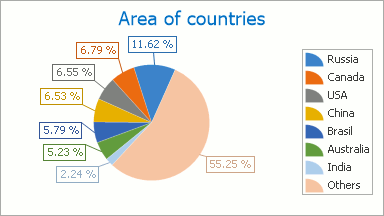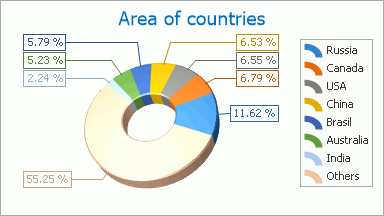XY-Diagram XY-Diagram 3D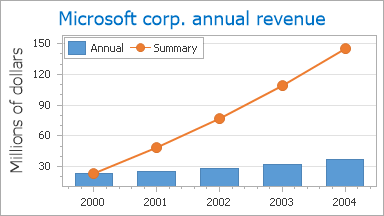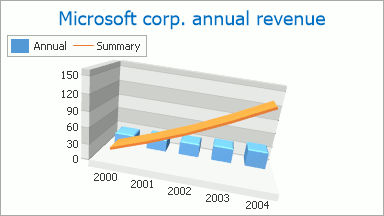Gantt Diagram Funnel Diagram 3D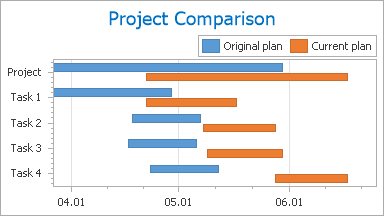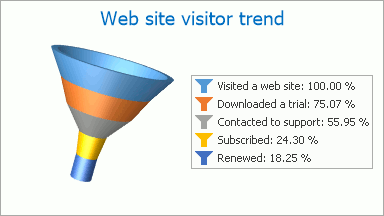Radar and Polar Diagrams Swift Plot Diagram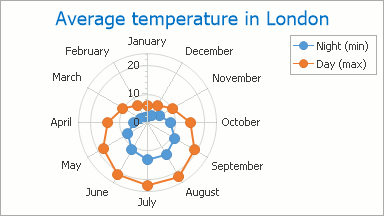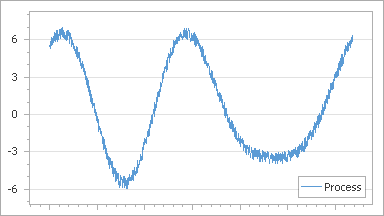To access almost any of the chart’s elements (excepting its series, titles and legend), you should first access the diagram itself. How this is done (both at design and runtime), is briefly described in the following section of this document: Accessing the Diagram.

## Accessing the Diagram

To access the diagram at design time, click your chart control, to select it. Then, in the Properties window, locate the WebChartControl.Diagram property, and expand it. You will see the list of available properties for this diagram type. Note that the set of these properties is dependent upon the diagram’s type.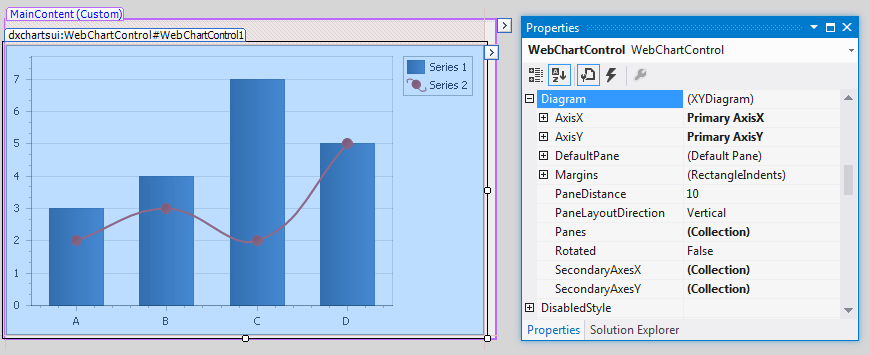At runtime, a diagram is represented by an instance of the Diagram class, which can be accessed via the WebChartControl.Diagram property. To access a diagram’s elements and properties, you should cast your diagram’s instance to the specific diagram’s type, as the following example demonstrates.

``````
// Create a new WebChartControl instance.
WebChartControl chartControl1= new WebChartControl();

// Create a series of a compatible view type,
// and add it to the chart's collection,
// so the diagram object shall not to be equal to null.
Series series1 = new Series("Line Series 1", ViewType.Line);